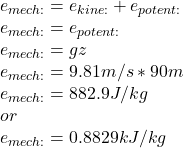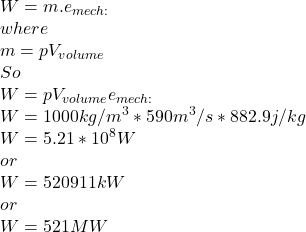Question

Consider a river flowing toward a lake at an average velocity of 3 m/s at a rate of 590 m3/s at a location 90 m above the lake surface. Determine the total mechanical energy of the river water per unit mass and the power generation potential of the entire river at that location. We take the density of water to be rho = 1000 kg/m3. The total mechanical energy of the river water per unit mass is kJ/kg. The power generation potential of the entire river at that location is kW.

1.Explanation:

Given data

Velocity v=3 m/s

Water volume flow rate V=590 m³/s

Water elevation z=90 m

Water density p=1000 kg/m³

First we need to determine the energy per unit mass

SoNow the power generation potential is: